UGC NET CS 2018 JUNE Paper-2

 Question 1
The definitions in an XML document are said to be when the tagging system and definitions in the DTD are all in compliance.
 A well-formed B reasonable C valid D logical
Web-Technologies       XML
Question 1 Explanation:
→ A XML document is said to be well- formed if it have correct syntax like tags are case sensitive, elements must have closing tags, elements must be properly nested etc.
→ But when when associate a well formed XML document with a DTD( in which we define the structure of a document) and if that XML document validates all the definitions defined in DTD then that XML document is called as “valid” XML document.
→ Always remember that a “valid” XML document is also a “well-formed” XML document but a “well-formed” XML document is not necessarily a “valid” XML document
→ In question, it is mentioned that XML document is validating the definitions in the DTD. so the answer is option (3).
 Question 2
Consider the JavaScript Code :
var y= ’’12”;
function f( )
{
var y=’’6”;
function g( )
{
}
g( );
}
f( );
If M is the number of alert dialog boxes generated by this JavaScript code and D1, D2, ...., DM represents the content displayed in each of the M dialog boxes, then :
 A M=3; D1 displays ”12”; D2 displays ”6”; D3 displays ”12”. B M=3; D1 displays ”6”; D2 displays ”12”; D3 displays ”6”. C M=2; D1 displays ”6”; D2 displays ”12”. D M=2; D1 displays ”12”; D2 displays ”6”.
Web-Technologies       Java-Script
Question 2 Explanation:
The JavaScript this keyword refers to the object it belongs to.
It has different values depending on where it is used:
In a method, this refers to the owner object.
Alone, this refers to the global object.
In a function, this refers to the global object.
In an event, this refers to the element that received the event.
Methods like call(), and apply() can refer this to any object.
There are two alert boxes in the javascript code.So two messages will be displayed.
First message, alert(this.y) here this.y is global variable whose value is 12 So first message is “12”.
Second message is alert(y) , here “y” local variable and value is 6 so second message displays value “6”
 Question 3
What is the output of the following JAVA program ?
class simple
{
public static void main(String[ ] args)
{
simple obj = new simple( );
obj.start( );
}
void start( )
{
long [ ] P= {3, 4, 5};
long [ ] Q= method (P);
System.out.print (P + P + P+”:”);
System.out.print (Q + Q + Q);
}
long [ ] method (long [ ] R)
{
R =7; return R;
}
} //end of class
 A 12 : 15 B 15 : 12 C 12 : 12 D 15 : 15
OOPS       JAVA
Question 3 Explanation:
First we will create the object of simple class.
By using object , we call the function start().
In the start() function definition, first statement is integer array with three elements.
long [ ] Q= method (P); Again function method(p) will be called.
In the definition of method function, we are changing the second element of array to value 7 and returning updated array to array Q.
We are passing address of P as argument to method so Modifications happened in the method automatically reflects to array P.
Both array P and Q consists of values {3,7,5}
The sum of the three values are 15
 Question 4
What is the output of the following ‘C’ program ? (Assuming little - endian representation of multi-byte data in which Least Significant Byte (LSB) is stored at the lowest memory address.)
#include
#include
/* Assume short int occupies two bytes of storage */
int main ( )
{
union saving
{
short int one;
char two;
};
union saving m;
m.two  = 5;
m.two  = 2;
printf(’’%d, %d, %d\n”, m.two , m.two , m.one);
}/* end of main */
 A 5, 2, 1282 B 5, 2, 52 C 5, 2, 25 D 5, 2, 517
Programming       Structure
Question 4 Explanation:
● m.two holds the value 5 and m.two holds the value 2 So it will print 5 and 2 values.
● Size of the short integer is 2 bytes. Saving is union variable, we will access one variable at time and only one memory location is shared among all the members.So the two values 5 and 2 (two bytes of the data) will store in little endian format in the variable m.one.
● Endianness is the sequential order in which bytes are arranged into larger numerical values when stored in memory or when transmitted over digital links.
● In big-endian format, whenever addressing memory or sending/storing words bytewise, the most significant byte—the byte containing the most significant bit—is stored first (has the lowest address) or sent first, then the following bytes are stored or sent in decreasing significance order,
● Little-endian format reverses this order: the sequence addresses/sends/stores the least significant byte first (lowest address) and the most significant byte last (highest address).
● First 5 will store in the lowest address and 2 will store next highest address.
● So the binary representation 5 and 2 in little endian format is 00000010 00000101
The binary number of the above is 517
 Question 5
Given below are three implementations of the swap( ) function in C++ :Which of these would actually swap the contents of the two integer variables p and q ?
 A (a) only B (b) only C (c) only D (b) and (c) only
Programming       Functions
Question 5 Explanation:
Module -(a) is call by value , so by using that code w can not swap the contents.
Module -(b) is call by reference , So the modification of content in the function reflects the changes in the main program. Swapping is done in the module -(b).
Module -(c) is passing addresses as parameters but in the functions definition , We are changing the addresses not the content So swapping of the values can’t be done.
 Question 6
​In Java, which of the following statements is/are True ?
S1 : The ‘final’ keyword applied to a class definition prevents the class from being extended through derivation.
S2 : A class can only inherit one class but can implement multiple interfaces.
S3 : Java permits a class to replace the implementation of a method that it has inherited. It is called method overloading.
 A S1 and S2 only B S1 and S3 only C S2 and S3 only D All of S1, S2 and S3
OOPS       JAVA
Question 6 Explanation:
● If a class has multiple methods having same name but different in parameters, it is known as Method Overloading.Method Overloading is a feature that allows a class to have more than one method having the same name, if their argument lists are different.So the option-3 is False.
● The final keyword in java is used to restrict the user. The java final keyword can be used in many context. Final can be: variable, method and class.
● A Java class can only extend one parent class. Multiple inheritance (extends) is not allowed. Interfaces are not classes, however, and a class can implement more than one interface.
 Question 7
Which of the following statements is/are True ?
P : C programming language has a weak type system with static types.
Q : Java programming language has a strong type system with static types.
 A P only B Q only C Both P and Q D Neither P nor Q
Programming       Storage-Classes
Question 7 Explanation:
→ A strongly typed language has stricter typing rules at compile time, which implies that errors and exceptions are more likely to happen during compilation. Most of these rules affect variable assignment, return values and function calling.
→ A weakly typed language has looser typing rules and may produce unpredictable results or may perform implicit type conversion at runtime
→ Java, C#, Ada and Pascal are sometimes said to be more strongly typed than C, a claim that is probably based on the fact that C supports more kinds of implicit conversions, and C also allows pointer values to be explicitly cast while Java and Pascal do not.
→ Java itself may be considered more strongly typed than Pascal as manners of evading the static type system in Java are controlled by the Java virtual machine's type system.
→ C# and VB.NET are similar to Java in that respect, though they allow disabling of dynamic type checking by explicitly putting code segments in an "unsafe context".
→ Pascal's type system has been described as "too strong", because the size of an array or string is part of its type, making some programming tasks very difficult
 Question 8
A graphic display system has a frame buffer that is 640 pixels wide, 480 pixels high and 1 bit of color depth. If the access time for each pixel on the average is 200 nanoseconds, then the refresh rate of this frame buffer is approximately :
 A 16 frames per second B 19 frames per second C 21 frames per second D 23 frames per second
Computer-Organization       Graphics
Question 8 Explanation:
Given data,
- Width (or) wide =640 pixels
- Height (or) High =480 pixels
- Color depth =1 bit/pixel
- Access time of each pixel on the average =200ns
- Refresh rate of frame buffer=?
Step-1: Graphic display system =640*480
=307200
Step-2: Memory required for Graphic display system =640*480*1 =307200
Step-3: Total screen access time = Memory required for Graphic display system * Access time of each pixel
= 307200*200 ns
= 61440000 ns
Step-4: Refresh rate of frame buffer per second=(10​ -9​ )/61440000
= 16.27604166 frames per second
[ Note: 10​ -9​ =1000000000 ]
 Question 9
Which of the following statements is/are True regarding the solution to the visibility problem in 3D graphics ?
S1 : The Painter’s algorithm sorts polygons by depth and then paints (scan - converts) each Polygon onto the screen starting with the most nearest polygon.
S2 : Backface Culling refers to eliminating geometry with back facing normals.
 A S1 only B S2 only C Both S1 and S2 D Neither S1 Nor S2
Computer-Organization       Graphics
Question 9 Explanation:
Visibility problem in 3D graphics
1. Painter's algorithm
-A depth sorting method
-Surfaces are sorted in the order of decreasing depth
-Surfaces are drawn in the sorted order, and overwrite the pixels in the frame buffer
-Subtle difference from depth buffer approach: entire face drawn
-Two problems:
1. It can be nontrivial to sort the surfaces
2. There can be no solution for the sorting order

2. Back Face Culling
-Back faces: faces of opaque object which are “pointing away” from viewer
-Back face culling – remove back faces (supported by OpenGL)
How to detect back faces
-If we find backface, do not draw, save rendering resources
-There must be other forward face(s) closer to eye
-F is face of object we want to test if backface
-P is a point on F
-Form view vector, V as (eye – P)
-N is normal to face F
3. View-Frustum Culling
-Remove objects that are outside the viewing frustum
-Done by 3D clipping algorithm (e.g. Liang-Barsky)
4. Ray Tracing
-Ray tracing is another example of image space method
-Ray tracing: Cast a ray from eye through each pixel to the world.
5. Z(Depth buffer algorithm)
 Question 10
Consider the matrixrepresenting a set of planar (2D) geometric transformations in homogeneous coordinates.
Which of the following statements about the matrix M is True ?
 A M represents first, a scaling of vector (2, 1) followed by translation of vector (1, 1) B M represents first, a translation of vector (1, 1) followed by scaling of vector (2, 1) C M represents first, a scaling of vector (3, 1) followed by shearing of parameters (−1, 1) D M represents first, a shearing of parameters (−1, 1) followed by scaling of vector (3, 1)
Engineering-Mathematics       Vectors
Question 10 Explanation:
Scale Matrix:
The scale matrix has all the same zeros as the identity matrix, but it doesn’t necessarily keep using the ones across the diagonal. You are trying to decide how to scale your coordinate, and you don’t want the default scale value to be 1. Here is the scale matrix:
[
Sx 0 0 0
0 Sy 0 0
0 0 Sz 0
0 0 0 1
]
Translation Matrix
The translation matrix looks the same as the identity matrix, but the last column is a little different. The last column applies an amount of change for the x, y, and z coordinates:
[
1 0 0 Tx
0 1 0 Ty
0 0 1 Tz
0 0 0 1
]
 Question 11
Assume the following regarding the development of a software system P:
- Estimated lines of code of P : 33, 480 LOC
- Average productivity for P : 620 LOC per person-month
- Number of software developers : 6
- Average salary of a software developer : 50,000 per month
If E, D and C are the estimated development effort (in person-months), estimated development time (in months), and estimated development cost (in Lac) respectively, then (E, D, C) =
 A (48, 8, 24) B (54, 9, 27) C (60, 10, 30) D (42, 7, 21)
Software-Engineering       Software-design
Question 11 Explanation:
- Estimated lines of code of P : 33480 LOC
- Average productivity for P : 620 LOC per person-month
- Number of software developers : 6
- Average salary of a software developer : 50000 per month
Step-1: Estimated development effort (in person-months)= 33480 /620
E=54
Step-2: Estimated development time (in months) =54/6
D= 9 months
Step-3: Estimated development cost (in Lac) = 50000*6*9
=27 lacs
 Question 12
Match the following in Software Engineering :A (a)-(ii), (b)-(iii), (c)-(iv), (d)-(i) B (a)-(iii), (b)- (i), (c)-(iv), (d)-(ii) C (a)-(iv), (b)- (i), (c)-(ii), (d)-(iii) D (a)-(iii), (b)- (iv), (c)-(i), (d)-(ii)
Software-Engineering       Software-requirements
Question 12 Explanation:
→ Coupling and Cohesion are used in software design. Cohesion measures strength of a module while coupling measures interdependency between modules.
→ Software cost estimation must be done more diligently throughout the project life cycle so that in the future there are fewer surprises and unforeseen delays in the release of a product.
→ Validation Technique→ Symbolic Execution
→ Software Requirements Definition→ Structured System Analysis
 Question 13
Which one of the following is not typically provided by Source Code Management Software ?
 A Synchronisation B Versioning and Revision history C Syntax highlighting D Project forking
Software-Engineering       Software-configuration-management
Question 13 Explanation:
Source Code Management Software is the management of changes to documents, computer programs, large web sites, and other collections of information. Changes are usually identified by a number or letter code, termed the "revision number", "revision level", or simply "revision".
→ Source Code Management Software includes
1. Synchronisation
2. Versioning and Revision history
3. Project forking
 Question 14
A software system crashed 20 times in the year 2017 and for each crash, it took 2 minutes to restart. Approximately, what was the software availability in that year ?
 A 96.9924% B 97.9924% C 98.9924% D 99.9924%
Software-Engineering       Software-Availability
Question 14 Explanation:
Software availability:​ It is a software used to ensure that systems are running and available most of the time. High availability is a high percentage of time that the system is functioning. It can be formally defined as (1 – (down time/ total time))*100%. Although the minimum required availability varies by task, systems typically attempt to achieve 99.999% (5-nines) availability. This characteristic is weaker than fault tolerance, which typically seeks to provide 100% availability, albeit with significant price and performance penalties.
Given data,
-Software crashed 20 times in the year 2017
-each crash=2 minutes to restart.
-Software availability=?
1 year=365 days = 365*1440 minutes =525600 minutes.
→ Crash time =20*2
=40 minutes
→ Software availability = (525600-40) / 525600
= 0.999924
= (0.999924 * 100)
= 99.9924 %
 Question 15
Match the 5 CMM Maturity levels/CMMI staged representations in List- I with their characterizations in List-II :A (a)-(iv), (b)-(v), (c)-(i), (d)-(iii), (e)-(ii) B (a)-(i), (b)-(ii), (c)-(iv), (d)-(v), (e)-(iii) C (a)-(v), (b)-(iv), (c)-(ii), (d)-(iii), (e)-(i) D (a)- (iv), (b)-(v), (c)-(ii), (d)-(iii), (e)-(i)
Question 15 Explanation:
Initial Stage: ​ There may not exist a plan or it may be abandoned.
Repeatable : ​ There’s a plan and people stick to it.
Defined : ​ The plan for a project comes from a template for plans.
Managed: ​ The plan uses processes that can be measured quantitatively.
Optimizing : Processes are improved quantitatively and continually.Question 16
Coupling is a measure of the strength of the interconnections between software modules. Which of the following are correct statements with respect to module coupling ?
P : Common coupling occurs when one module controls the flow of another module by passing it information on what to do.
Q : In data coupling, the complete data structure is passed from one module to another through parameters.
R : Stamp coupling occurs when modules share a composite data structure and use only parts of it.
 A P and Q only B P and R only C Q and R only D All of P, Q and R
Software-Engineering       Software-process-models
Question 16 Explanation:
Common Coupling:​ Common coupling occurs if two modules share same global data.
Data Coupling :​ Data coupling occurs when two modules communicate using elementary data items that are passed as parameters between two modules.
Stamp Coupling​ : Stamp coupling occurs if two modules communicate using composite items such as records in Pascal or structure in C
 Question 17
A software design pattern often used to restrict access to an object is :
 A adapter B decorator C delegation D proxy
Software-Engineering       Software-design
Question 17 Explanation:
Proxy pattern:​ a class functioning as an interface to another thing. In the proxy, extra functionality can be provided, for example caching when operations on the real object are resource intensive, or checking preconditions before operations on the real object are invoked.
→ For the client, usage of a proxy object is similar to using the real object, because both implement the same interface.
→ Proxy pattern solve the access to an object should be controlled and functionality should be provided when accessing an object.
→ When accessing sensitive objects, for example, it should be possible to check that clients have the needed access rights.
 Question 18
Reasons to re-engineer a software include :
P : Allow legacy software to quickly adapt to the changing requirements
R : Improve software maintainability
S : Allow change in the functionality and architecture of the software
 A P, R and S only B P and R only C P, Q and S only D P, Q and R only
Software-Engineering       Software-design
Question 18 Explanation:
→ Software re-engineering is the examination and alteration of a system to reconstitute it in a new form.​ It is done to improve the maintainability of the software.
→ Software reengineering encompasses inventory analysis, document restructuring, reverse engineering, program and data restructuring, and forward engineering. The intent of these activities is to create versions of existing programs that exhibit higher quality and better Maintainability.
A software reengineering process model:Question 19
Which of the following is not a key strategy followed by the clean room approach to software development ?
 A Formal specification B Dynamic verification C Incremental development D Statistical testing of the system
Software-Engineering       Software-Development
Question 19 Explanation:
The basic principles of the clean room process are
1. Software development based on formal methods: Software tool support based on some mathematical formalism includes model checking, process algebras, and Petri nets. The Box Structure Method might be one such means of specifying and designing a software product. Verification that the design correctly implements the specification is performed through team review, often with software tool support.
2. Incremental implementation under statistical quality control Cleanroom development uses an iterative approach, in which the product is developed in increments that gradually increase the implemented functionality. The quality of each increment is measured against pre-established standards to verify that the development process is proceeding acceptably. A failure to meet quality standards results in the cessation of testing for the current increment, and a return to the design phase.
3. Statistically sound testing
Software testing in the cleanroom process is carried out as a statistical experiment. Based on the formal specification, a representative subset of software input/output trajectories is selected and tested. This sample is then statistically analyzed to produce an estimate of the reliability of the software, and a level of confidence in that estimate.
 Question 20
Which of the following statements is/are True ?
P : Refactoring is the process of changing a software system in such a way that it does not alter the external behavior of the code yet improves the internal architecture.
Q : An example of refactoring is adding new features to satisfy a customer requirement discovered after a project is shipped.
 A P only B Q only C Both P and Q D Neither P nor Q
Software-Engineering       Software-design
Question 20 Explanation:
→ Refactoring allows a software engineer to improve the internal structure of a design (or source code) without changing its external functionality or behavior of the code yet improves the internal structure. In essence, refactoring can be used to improve the efficiency, readability, or performance of a design or the code that implements a design.
→ It is a disciplined way to clean up code [and modify/simplify the internal design] that minimizes the chances of introducing bugs. In essence, when you refactor you are improving the design of the code after it has been written.
Note: It won’t add any new featuring to satisfy a customer requirement. So, statement Q is false.
 Question 21
The solution of the recurrence relation T(m)=T(3m/4)+1 is :
 A θ(lg m) B θ(m) C θ(mlg m) D θ(lglg m)
Algorithms       Asymptotic-Complexity
Question 21 Explanation:
Using Masters Method:
a=1, b=4/3, k=0, p=0
Here, a = b​ k
So, T(m) = n log a/ log b log p+1 n
T(m) = θ(log m)
 Question 22
Consider the array A=<4, 1, 3, 2, 16, 9, 10, 14, 8, 7>. After building heap from the array A, the depth of the heap and the right child of max-heap are__________ and __________ respectively. (Root is at level 0).
 A 3, 14 B 3, 10 C 4, 14 D 4, 10
Data-Structures       Heap-Tree
Question 22 Explanation:
A heap is a MAX-Heap if all the root nodes(parent nodes) have maximum value.
Step 1: Since a heap is a almost complete binary tree so build a heap first.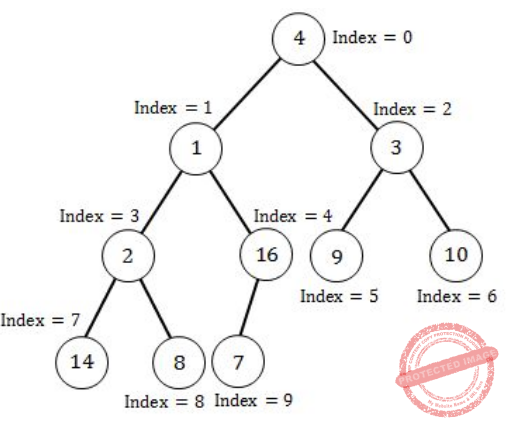Step 2: Since the above heap is not a max heap so to convert a heap into max-heap start applying max- heapify operation from the largest index parent node(node having 1 or more children).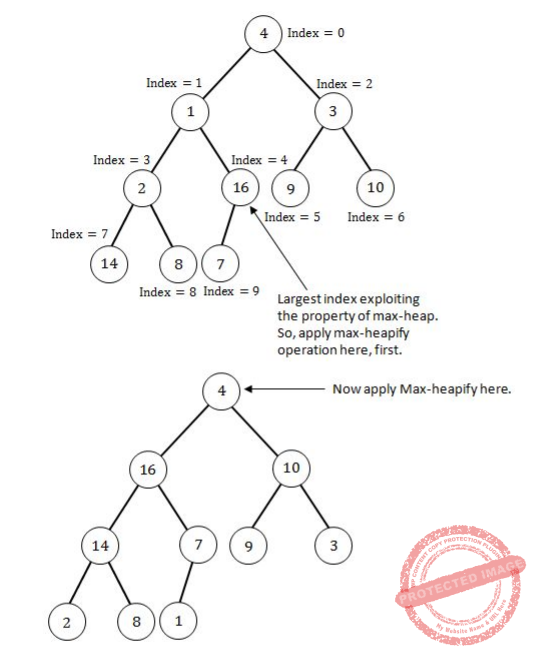The above Heap is the max-heap where each root node have maximum value. Now depth of the Max-heap is 3 and right child of the Root node is 10.
 Question 23
A hash function h defined h(key)=key mod 7, with linear probing, is used to insert the keys 44, 45, 79, 55, 91, 18, 63 into a table indexed from 0 to 6. What will be the location of key 18 ?
 A 3 B 4 C 5 D 6
Data-Structures       Hashing
Question 23 Explanation:
h(key)=key mod 7 is the given hash function and it is mentioned that linear probing is used.
h(44)=44 mod 7 ⇒ 2
h(45)=45 mod 7 ⇒ 3
h(79)=79 mod 7 ⇒ 2 (collision occurred)
h(79)=(79+1) mod 7 ⇒ 3 (collision occurred)
h(79)=(79+2) mod 7 ⇒ 4
h(55)=55 mod 7 ⇒ 6
h(91)=91 mod 7 ⇒ 0
h(18)=18 mod 7 ⇒ 4 (collision occurred)
h(79)=(18+1) mod 7 ⇒ 5
h(63)=63 mod 7 ⇒ 0 (collision occurred)
h(63)=(63+1) mod 7 ⇒ 1
Now the array contain keys 44, 45, 79, 55, 91, 18, 63 at locationsQuestion 24
Which of the following algorithms solves the single-source shortest paths ?
 A Prim’s algorithm B Floyd - Warshall algorithm C Johnson’s algorithm D Dijkstra’s algorithm
Algorithms       Greedy-approach
Question 24 Explanation:
Dijkstra’s algorithm is a Single source shortest path algorithm used to find the shortest path using Greedy approach.
 Question 25
A text is made up of the characters A, B, C, D, E each occurring with the probability 0.08, 0.40, 0.25, 0.15 and 0.12 respectively. The optimal coding technique will have the average length of :
 A 2.4 B 1.87 C 3 D 2.15
Algorithms       Greedy-approach
Question 25 Explanation:Step 1: Select two characters with smallest probabilities and merge them.Step 2: Select two characters from Step 1 with smallest probabilities and merge them.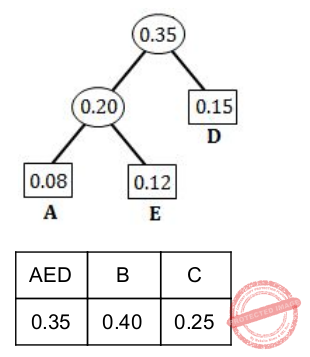Step 3: Select two characters (from Step 2) with smallest probabilities and merge them.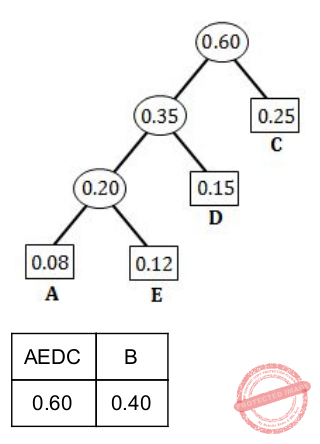Step 4: Merge the remaining two probabilities.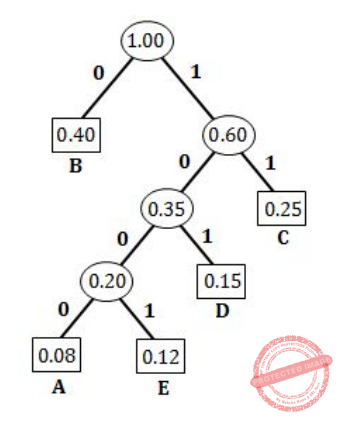A = 1000 (4-bits)
E = 1001 (4-bits)
D = 101 (3-bits)
C = 11 (2-bits)
B = 0 (1-bit)
Average length = ((0.08×4)+(0.12×4)+(0.15×3)+(0.25×2)+(0.40×1)) / (0.08+0.12+0.15+0.25+0.40)
=2.15/1.0
= 2 .15
 Question 26
A binary search tree in which every non-leaf node has non-empty left and right subtrees is called a strictly binary tree. Such a tree with 19 leaves :
 A cannot have more than 37 nodes B has exactly 37 nodes C has exactly 35 nodes D cannot have more than 35 nodes
Data-Structures       Binary-search-tree
Question 26 Explanation:
L = I(n-1)+ 1
Where, L= Number of leaf nodes
n= N-ary tree
I = Number of intermediate nodes
Now, 19 = I(2-1)+1
19 = 2I-I+1
19 = I+1
I = 18
Total Number of nodes in a tree = Number of Intermediate nodes+Number of Leaf nodes
Total Number of nodes in a tree = 19+18
Total Number of nodes in a tree = 37
 Question 27
Match the following with respect to algorithm paradigms :A (a)-(iv),(b)-(i), (c)-(iii), (d)-(ii) B (a)-(iv),(b)-(iii), (c)-(i), (d)-(ii) C (a)-(iii),(b)-(iv), (c)-(ii), (d)-(i) D (a)-(iv),(b)-(iii), (c)-(ii), (d)-(i)
Algorithms       Dynamic-Programming-and-Greedy-approach
Question 27 Explanation:
8-Queens problem use Backtracking
Single-Source shortest paths use Greedy approach
STRASSEN’s Matrix multiplication use Divide and conquer technique
Optimal binary search trees use Dynamic programming
 Question 28
The maximum number of comparisons needed to sort 9 items using radix sort is (assume each item is 5 digit octal number) :
 A 45 B 72 C 360 D 450
Algorithms       Sorting
Question 28 Explanation:
Total sort items=9
Octal number having→ 5 digits
The octal number system base value= 8
The maximum number of comparison=(number of items)*(radix)*(number of digits)
= 9*5*8
= 360
 Question 29
A 5-ary tree is tree in which every internal node has exactly 5 children. The number of left nodes in such a tree with 8 internal nodes will be :
 A 30 B 33 C 45 D None of the above
Data-Structures       Trees
Question 29 Explanation:
L = I(n-1)+ 1
Where, L= Number of leaf nodes
n= N-ary tree
I = Number of intermediate nodes
Now, L =8(5-1)+1
L =8(4)+1
L =33
Total Number of nodes in a tree = Number of Intermediate nodes+Number of Leaf nodes
Total Number of nodes in a tree = 8+33
Total Number of nodes in a tree = 41
 Question 30
Consider a Boolean function of ‘n’ variables. The order of an algorithm that determines whether the Boolean function produces a output 1 is :
 A Logarithmic B Linear C Quadratic D Exponential
Digital-Logic-Design       Boolean-Function
Question 30 Explanation:Set 1 have “n” variables and each variable in set 1 can be mapped with one boolean value in
set 2 i.e. each variable in set 1 have 2 choices and we have “n” such variables in set 1.
→ So, total number of choices we get maximum 2​ n​ . It means exponential.
 Question 31
Two finite state machines are said to be equivalent if they :
 A Have the same number of edges B Have the same number of states C Recognize the same set of tokens D Have the same number of states and edges
Theory-of-Computation       Finite-Automata
Question 31 Explanation:
We can call two finite state machines as equivalent if and only if the accept the same language.
 Question 32
The finite state machine given in figure below recognizes :A any string of odd number of a’s B any string of odd number of b’s C any string of even number of a’s and odd number of b’s D any string of odd number of a’s and odd number of b’s
Theory-of-Computation       Finite-Automata
Question 32 Explanation:
The language accepted by above finite automata is:
L = {ab,ba,ababab, bababa,..........}
Here L is the language containing odd number of a’s and odd number of b’s.
 Question 33
A pushdown automata behaves like a Turing machine when the number of auxiliary memory is :
 A 0 B 1 C 1 or more D 2 or more
Theory-of-Computation       Turing-machines
Question 33 Explanation:
→ If we have two stacks then the stacks can act as a queue. Since a pushdown automata have a single stack as its memory device so if we have 1 more stack then the pushdown automata can be made to act as a turing machine.
→ So, total of 2 or more auxiliary memory are needed to make a pushdown automata behaves like a Turing machine.
 Question 34
Pushdown automata can recognize language generated by .
 A Only context free grammar B Only regular grammar C Context free grammar or regular grammar D Only context sensitive grammar
Theory-of-Computation       Push-Down-Automata
Question 34 Explanation:
Let A➝ aA | ∈ ; Here A is a regular grammar which can be accepted by the pushdown automata
B➝ aBb | ab ; Here B is a Context free grammar which can be accepted by the pushdown automata
 Question 35
To obtain a string of n Terminals from a given Chomsky normal form grammar, the number of productions to be used is :
 A 2n−1 B 2n C n+1 D n​ 2
Theory-of-Computation       Context-Free-Grammar
Question 35 Explanation:
If all the productions of a grammar are in the form A➝BC OR A➝a then that grammar is said to be in Chomsky normal form.
Example:
S → A B
A → a
B → b
Derive string “ab” from above grammar.
Detailed explanation for example:Using formula 2 n − 1 ; where n = length of string
2 (2) − 1 = 3
Hence the answer is option(1)
 Question 36
Consider the following two Grammars :
G1 : S → SbS|a
G2 : S → aB|ab, A→GAB|a, B→ABb|b
Which of the following option is correct ?
 A Only G1 is ambiguous B Only G2 is ambiguous C Both G1 and G2 are ambiguous D Both G1 and G2 are not ambiguous
Compiler-Design       Ambiguous-and-Unambiguous-Grammar
Question 36 Explanation:
A grammar is said to be ambiguous if we get two different parse trees using either Leftmost derivation only or Rightmost derivation only.
To generate string “ababa” using G1 grammar the two different parse tree possible are:Question 37
Context sensitive language can be recognized by a :
 A Finite state machine B Deterministic finite automata C Non-deterministic finite automata D Linear bounded automata
Theory-of-Computation       Context-sensitive-language
Question 37 Explanation:
Context sensitive languages can be recognized by the Linear bounded automata.
L= { a n b n c n | where n > 0 }
is a context sensitive language and cannot be recognized by Finite state machine, Deterministic finite automata, Non-deterministic finite automata but L can be recognized by Linear Bounded Automata.
So Context Sensitive Language can be recognized by Linear Bounded Automata.
 Question 38
The set A={ 0​ n​ 1​ n​ 2​ n​ | n=1, 2, 3, ......... } is an example of a grammar that is :
 A Context sensitive B Context free C Regular D None of the above
Theory-of-Computation       Context-Sensitive-Grammar
Question 38 Explanation:
→ For above set we need infinite tape so this can’t be an example of Regular Grammar.
→ In case of Context Free grammar we have a stack only in which we can push 0’s as they come as input and can POP 0’s as 1’s come as input but when 2’s comes as input string we have NULL at the top of the stack so this Grammar can’t be a context free grammar.
→ Since in case of Context sensitive grammar we have linear bounded automata and a read/write head which can move backward and upward so this grammar can be accepted by Linear Bounded Automata. So this grammar is a Context Sensitive Grammar.
 Question 39
A bottom-up parser generates :
 A Leftmost derivation in reverse B Right-most derivation in reverse C Left-most derivation D Right-most derivation
Compiler-Design       Parsers
Question 39 Explanation:
A bottom-up parser uses Right-most derivation in reverse order to decide “What to Reduce”.
 Question 40
Consider the following statements( ) :
S1 : There exists no algorithm for deciding if any two Turing machines M1 and M2 accept the same language.
S2 : The problem of determining whether a Turing machine halts on any input is undecidable.
Which of the following options is correct ?
 A Both S1 and S2 are correct B Both S1 and S2 are not correct C Only S1 is correct D Only S2 is correct
Theory-of-Computation       Turing-machines
Question 40 Explanation:
→ Checking if any two Turing machines M1 and M2 accept the same language or not is the equivalence problem. And equivalence problem of turing machines is Undecidable. So Statement S1 is correct.
→ Halting problem of a turing machine is undecidable because if a turing machine accepts a string then it will halt but if a turing machine does not accept a string then it may or may not halt(can enter infinite loop). So statement S2 is also correct.
 Question 41
A slotted ALOHA network transmits 200-bit frames using a shared channel with a 200 Kbps bandwidth. Find the throughput of the system, if the system (all stations put together) produces 250 frames per second :
 A 49 B 368 C 149 D 151
Computer-Networks       Access-Control-Methods
Question 41 Explanation:
Step-1: Given data,
Slotted ALOHA transmits=200 bit frames
Bandwidth=200 Kbps
System produces=250 frames per second
Throughput=?
Step-2: Pure ALOHA formula S= G*e​ -2G
Slotted ALOHA formula S=G*e​ -G
Frame transmission time=200/200 kbps=1ms
Here, G=1⁄4 and S=G*e​ -G
=0.195 (or) 19.5%
System produces=250*0.195
=48.75
 Question 42
The period of a signal is 100 ms. Its frequency is .
 A 1003 Hertz B 10​ −2​ KHz C 10​ −3​ KHz D 105 Hertz
Question 42 Explanation:
First we change 100 ms to seconds, and then we calculate the frequency from the period (1 Hz = 10​ −3​ kHz).
100 ms = 100 × 10 −3 s = 10 −1 s
f =1/T=(1/10) −1Hz= 10 Hz = 1 0 × 10 −3 kHz = 10 −2 kHz
 Question 43
The dotted-decimal notation of the following IPV4 address in binary notation is .
10000001 00001011 00001011 11101111
 A 111.56.45.239 B 129.11.10.238 C 129.11.11.239 D 111.56.11.239
Computer-Networks       IPv4-an-Fragmentation
Question 43 Explanation:
10000001 00001011 00001011 11101111
Since the IP address is of 32 bits and is divided in 4 parts of size 8-bits and then perform simple binary to decimal conversion.Question 44
Which of the following statements are true ?
(a) Advanced Mobile Phone System (AMPS) is a second generation cellular phone system.
(b) IS - 95 is a second generation cellular phone system based on CDMA and DSSS.
(c) The Third generation cellular phone system will provide universal personnel communication.
 A (a) and (b) only B (b) and (c) only C (a), (b) and (c) D (a) and (c) only
Question 44 Explanation:
(a) FALSE: AMPS is a first-generation cellular technology that uses separate frequencies, or "channels", for each conversation (see frequency-division multiple access (FDMA)). It therefore required considerable bandwidth for a large number of users. In general terms, AMPS was very similar to the older "0G" Improved Mobile Telephone Service, but used considerably more computing power in order to select frequencies, hand off conversations to PSTN lines, and handle billing and call setup.
(b) TRUE: IS - 95 is a second generation cellular phone system based on CDMA and DSSS.
(c) TRUE: The Third generation cellular phone system will provide universal personnel communication.
 Question 45
Match the following symmetric block ciphers with corresponding block and key sizes :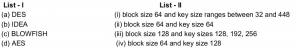A (a)-(iv), (b)-(ii), (c)-(i), (d)-(iii) B (a)-(ii), (b)-(iv), (c)-(i), (d)-(iii) C (a)-(ii), (b)-(iv), (c)-(iii), (d)-(i) D (a)-(iv), (b)-(ii), (c)-(iii), (d)-(i)
Computer-Networks       Network-Security
Question 45 Explanation:
→ Data Encryption Standard(DES), which uses symmetric key method for encryption of data. It uses block size 64 and key size 128 for encryption.
→ International Data Encryption Algorithm (IDEA), originally called Improved Proposed Encryption Standard (IPES), is a symmetric-key block cipher. It uses block size 64 and key size 128.
→ Blowfish is a symmetric-key block cipher used for a large number of cipher suites and encryption products. Blowfish provides a good encryption rate in software and no effective cryptanalysis of it has been found to date. It uses block size 64 and key size ranges between 32 and 448.
→ Advanced Encryption Standard(AES), which uses symmetric key method for encryption of data. It uses block size 128 and key sizes 128, 192, 256
*****It’s worthy to remember below table.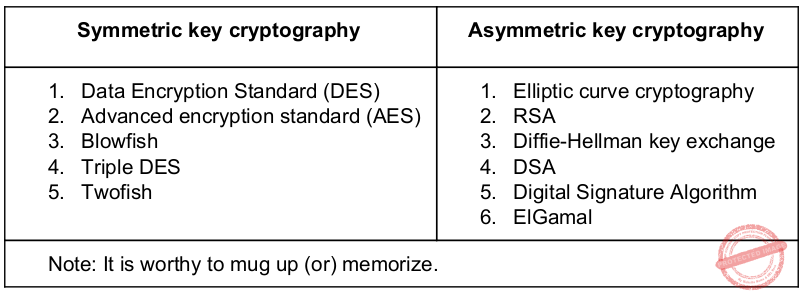Question 46
Which of the following statements are true ?
(a) Three broad categories of Networks are
(i) Circuit Switched Networks
(ii) Packet Switched Networks
(iii) Message Switched Networks
(b) Circuit Switched Network resources need not be reserved during the set up phase.
(c) In packet switching there is no resource allocation for packets.
 A (a) and (b) only B (b) and (c) only C (a) and (c) only D (a), (b) and C
Computer-Networks       Switching
Question 46 Explanation:
→ There are three broad categories of Networks Circuit Switched Networks, Packet Switched Networks, Message Switched Networks.
→ In circuit switching resources are reserved from Setup phase to data transmission phase.
→ When data transmission completes only then the reserved resources are released.
→ Since in packet switching each packet follows different path to reach the destination so no resource reservation is done in case of packet switching.
 Question 47
​In Challenge-Response authentication the claimant .
 A Proves that she knows the secret without revealing it B Proves that she doesn’t know the secret C Reveals the secret D Gives a challenge
Computer-Networks       Network-Security
Question 47 Explanation:
→ Challenge-Response authentication is a family of protocols in which one party presents a question ("challenge") and another party must provide a valid answer ("response") to be authenticated.
→ The simplest example of a challenge–response protocol is password authentication, where the challenge is asking for the password and the valid response is the correct password.
→ A more interesting challenge–response technique works as follows. Say, Bob is controlling access to some resource. Alice comes along seeking entry. Bob issues a challenge, perhaps "52w72y". Alice must respond with the one string of characters which "fits" the challenge Bob issued. The "fit" is determined by an algorithm "known" to Bob and Alice. (The correct response might be as simple as "63x83z" (each character of response one more than that of challenge), but in the real world, the "rules" would be much more complex.) Bob issues a different challenge each time, and thus knowing a previous correct response (even if it isn't "hidden" by the means of communication used between Alice and Bob) is of no use.
 Question 48
Decrypt the message “WTAAD” using the Caesar Cipher with key=15.
 A LIPPS B HELLO C OLLEH D DAATW
Computer-Networks       Network-Security
Question 48 Explanation: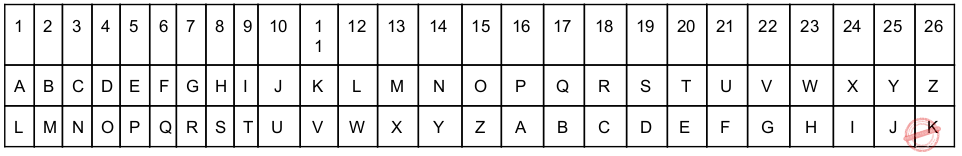We decrypt one character at a time. Each character is shifted 15 characters up Letter W is decrypted to H shifted 15 characters up. Letter W is decrypted to H. Letter T is decrypted to E.The first A is decrypted to L. The second A is decrypted to L And finally D is The second A is decrypted to L. And, finally, D is decrypted to O.The plain text is HELLO
 Question 49
To guarantee correction of upto t errors, the minimum Hamming distance dmin in a block code must be .
 A t+1 B t−2 C 2t−1 D 2t+1
Computer-Networks       Error-Control-Methods
Question 49 Explanation:
For detect t-bit errors a hamming distance of t+1 bit is needed but to correct the t-bit error the hamming distance of 2t+1 bit is needed.
 Question 50
Encrypt the Message “HELLO MY DEARZ” using Transposition Cipher withA HLLEO YM AEDRZ B EHOLL ZYM RAED C ELHL MDOY AZER D ELHL DOMY ZAER
Computer-Networks       Network-Security
Question 50 Explanation:
Step-1: According to key we have to divide into number of character blocks.
Here, key size=4. So, character block size is 4.
Step-2: Remove the spaces in the message and write into sequential order.Step-3: Get cipher text according to ascending order is ELHL MDOY AZER.
 Question 51
At a particular time of computation, the value of a counting semaphore is 10. Then 12 P operations and “x” V operations were performed on this semaphore. If the final value of semaphore is 7, x will be :
 A 8 B 9 C 10 D 11
Operating-Systems       Semaphores
Question 51 Explanation:
Initial value of counting semaphore is 10. And when a process enters the critical section it decreases the value of counting semaphore using P operation and when a process leaves the critical section it increases the value of counting semaphore using V operation.
So here 12P (subtraction) and x(addition) operation are given. And after performing P(subtraction) and V(addition) the value of counting semaphore is 7.
7 = 10-12+x
x=9
 Question 52
In a paged memory, the page hit ratio is 0.40. The time required to access a page in secondary memory is equal to 120 ns. The time required to access a page in primary memory is 15 ns. The average time required to access a page is .
 A 105 B 68 C 75 D 78
Operating-Systems       Memory-Management
Question 52 Explanation:
Average time to access a page = page hit ratio(time required to access a page in primary memory)+ page miss ratio(time required to access a page in primary memory+time required to access a page in secondary memory)
Average time to access a page = 0.40(15)+ 0.60(120)
Average time to access a page = 6+72
Average time to access a page = 78
 Question 53
In a multi-user operating system, 30 requests are made to use a particular resource per hour, on an average. The probability that no requests are made in 40 minutes, when arrival pattern is a poisson distribution, is .
 A e​ -15 B 1-e​ -15 C 1-e​ -20 D e​ -20
Engineering-Mathematics       Probability
Question 53 Explanation:
→ In probability theory, a Poisson process is a stochastic process that counts the number of events and the time points at which these events occur in a given time interval.
→ The time between each pair of consecutive events has an exponential distribution with parameter λ and each of these inter-arrival times is assumed to be independent of other inter-arrival times.
→ λ = (40*30)/60
=20
P(T>40min)= 1-P(T ≤ 40 min)
=1-(1-e​ -40 min / λ​ )
=1-(1-e​ -40 min / 20​ )
= e​ -20
 Question 54
Normally user programs are prevented from handling I/O directly by I/O instructions in them. For CPUs having explicit I/O instructions, such I/O protection is ensured by having the I/O instructions privileged. In a CPU with memory mapped I/O, there is no explicit I/O instruction. Which one of the following is true for a CPU with memory mapped I/O ?
 A I/O protection is ensured by operating system routines. B I/O protection is ensured by a hardware trap. C I/O protection is ensured during system configuration. D I/O protection is not possible.
Computer-Organization       Isolated-vs-Memory-mapped-I/O
Question 54 Explanation:
→ I/O protection can be ensured by operating system. Because all the user application are not modified by user mode. Those are sent to kernel mode as a system calls.
→ Normally user programs are prevented from handling I/O directly by I/O instructions in them , For CPUs having explicit I/O instructions, such I/O protection is ensured by having the I/O instruction privileged.
 Question 55
Which UNIX/Linux command is used to make all files and sub-directories in the directory “progs” executable by all users ?
 A chmod− R a+x progs B chmod −R 222 progs C chmod−X a+x progs D chmod −X 222 progs
Operating-Systems       UNIX-Operating-System
Question 55 Explanation:
Here, observe 2 key points is
1. All files and their subdirectories
2. Execute by all users
Step-1: Normally any file consists of 3 categories
1. Owner
2. Group
3. Others
Step-2: Every category is represented in 3 accesses.
1. Read(Octal value 4)
2. Write(Octal value 2)
3. Execute(Octal value 1)
Step-3: To change user permission, we are using command is chmod.
Chmod syntax: chmod permissions filename
1. All files and their subdirectories (using recursive command ‘R’)
2. Execute by all users(using ‘a+x’ subcommand. ‘a’ is nothing but all and ‘+x’ is execute permissions)
Actual command required: chmod− R a+x progs
 Question 56
Which of the following statements are true ?
(a) External Fragmentation exists when there is enough total memory space to satisfy a request but the available space is contiguous.
(b) Memory Fragmentation can be internal as well as external.
(c) One solution to external Fragmentation is compaction.
 A (a) and (b) only B (a) and (c) only C (b) and (c) only D (a), (b) and (c)
Operating-Systems       Memory-Management
Question 56 Explanation:
External Fragmentation exists when there is enough total memory space to satisfy a request but the available space is ​ not ​ contiguous.
Yes, it is true that memory Fragmentation can be internal as well as external.
Yes, compaction is a solution to external Fragmentation.
 Question 57
Page information in memory is also called as Page Table. The essential contents in each entry of a page table is/are .
 A Page Access information B Virtual Page number C Page Frame number D Both virtual page number and Page Frame Number
Operating-Systems       Memory-Management
Question 57 Explanation:
→ For every page table it contains page frame number.
→ Virtual page number can represents index in the page table to get the page frame number.
 Question 58
Consider a virtual page reference string 1, 2, 3, 2, 4, 2, 5, 2, 3, 4. Suppose LRU page replacement algorithm is implemented with 3 page frames in main memory. Then the number of page faults are .
 A 5 B 7 C 9 D 10
Operating-Systems       Page-Replacement-algorithm
Question 58 Explanation:So total number of page faults are 7.
 Question 59
Consider the following three processes with the arrival time and CPU burst time given in milliseconds :The Gantt Chart for preemptive SJF scheduling algorithm is
 A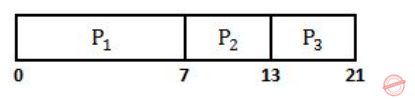BC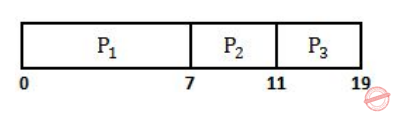DOperating-Systems       Process-Scheduling
Question 59 Explanation:Question 60
In which of the following scheduling criteria, context switching will never take place ?
 A ROUND ROBIN B Preemptive SJF C Non-preemptive SJF D Preemptive priority
Operating-Systems       Context-Switching
Question 60 Explanation:
ROUND ROBIN : In this because of time quantum context switching will take place
Preemptive SJF : In this context switching will take place if a smaller burst time process arrives in ready queue.
Non-preemptive SJF: Since it is non-preemptive SJF scheduling so a process will leave CPU only when it is completely executed.
Preemptive priority : In this Context switching will take place if a process with higher priority enters the ready queue.
 Question 61
In RDBMS, which type of Join returns all rows that satisfy the join condition ?
 A Inner Join B Outer Join C Semi Join D Anti Join
Database-Management-System       Relational-Algebra
Question 61 Explanation:
Inner Join : Inner join combines two tables having a common attributes. While combining it only join the rows of two tables having same value in common attribute. So in that way inner join return the records having matching values in both the tables.
 Question 62
Consider a relation book(title, price) which contains the titles and prices of different books. Assuming that no two books have the same price, what does the following SQL query list ?
SELECT title
FROM book AS B
WHERE(SELECT COUNT(*) FROM book AS T WHERE T.price > B.price) < 7
 A Titles of the six most expensive books. B Title of the sixth most expensive books. C Titles of the seven most expensive books. D Title of the seventh most expensive books.
Database-Management-System       SQL
Question 62 Explanation:
→ SQL query, which results titles of the 7 most expensive books.
→ The where clause of outer query will be true for 7 most expensive books.
Note: All aggregate functions except count(*) ignore NULL values in their input collection.
 Question 63
In a Hierarchical database, a hashing function is used to locate the .
 A Collision B Root C Foreign Key D Records
Database-Management-System       Indexing
Question 63 Explanation:
→ A hierarchical database model is a data model in which the data are organized into a tree-like structure. The data are stored as records which are connected to one another through links. A record is a collection of fields, with each field containing only one value. The type of a record defines which fields the record contains.
→ The hierarchical database model mandates that each child record has only one parent, whereas each parent record can have one or more child records.
→ In order to retrieve data from a hierarchical database the whole tree needs to be traversed starting from the root node.
→ In a Hierarchical database, a hashing function is used to locate the root node.
 Question 64
Relations produced from E-R Model will always be in .
 A 1 NF B 2 NF C 3 NF D 4 NF
Database-Management-System       ER-Model
Question 64 Explanation:
Relations produced from E-R Model will always be in 3NF.
 Question 65
Consider the following schedules involving two transactions.
S1: r1(X) ; r1(Y) ; r2(X) ; r2(Y) ; w2(Y) ; w1(X)
S2 : r1(X) ; r2(X) ; r2(Y) ; w2(Y) ; r1(Y) ; w1(X)
Which one of the following statements is correct with respect to above ?
 A Both S1 and S2 are conflict serializable. B Both S1 and S2 are not conflict serializable. C S1 is conflict serializable and S2 is not conflict serializable. D S1 is not conflict serializable and S2 is conflict serializable.
Database-Management-System       Transactions
Question 65 Explanation:Question 66
For a database relation R(a, b, c, d) where the domains of a, b, c and d include only atomic values, and only the following functional dependencies and those that can be inferred from them hold :
a → c
b → d
The relation is in .
 A First normal form but not in second normal form B Second normal form but not in third normal form C Third normal form D BCNF
Database-Management-System       Functional-Dependency
Question 66 Explanation:
Primary key of given relation is “ab” And there is a partial dependency exist in given FD’s so the given relation is in 1NF but not in second normal form.
 Question 67
A many-to-one relationship exists between entity sets r1 and r2. How will it be represented using functional dependencies if Pk(r) denotes the primary key attribute of relation r?
 A Pk(r1 ) → Pk(r2 ) B Pk(r2 ) → Pk(r1 ) C Pk(r2 ) → Pk(r1 ) and Pk(r1 ) → Pk(r2 ) D Pk(r2 ) → Pk(r1 ) or Pk(r1 ) → Pk(r2 )
Database-Management-System       Functional-Dependency
Question 67 Explanation:Here we have a many to one relationship between between Set(r1) and set(r2).
→ Elements of set(r2) can’t identify elements of sert(r1) because one value element in set(r2) is pointing to more than one element of set(r1).
→ So we can’t say Pk(r2 ) → Pk(r1) but elements of set(r1) are pointing to exactly one element of set(r2) so we can say that Pk(r2 ) → Pk(r1 ) because r1 is uniquely identifying r2.
 Question 68
Database systems that store each relation in a separate operating system file may use the operating system’s authorization scheme, instead of defining a special scheme themselves. In this case, which of the following is false ?
 A The administrator enjoys more control on the grant option. B It is difficult to differentiate among the update, delete and insert authorizations. C Cannot store more than one relation in a file D Operations on the database are speeded up as the authorization procedure is carried out at the operating system level.
Database-Management-System       SQL
Question 68 Explanation:
When the Database system will store each relation in seperate operating system file then it will become difficult for the administrator to differentiate among the update, delete and insert authorizations and also to keep track of grant options becomes difficult which is a overhead.
So option 1 is clearly false and option 2 is true.
Option 3: In question it is mentioned that each relation is stored in a separate operating system file. So option 3 is true.
Option 4 : Since for each relation there is a seperate operating system file which may use the operating system’s authorization scheme. So Operations on the database are speeded up as the authorization procedure is carried out at the operating system level.
 Question 69
Let R1(a,b,c) and R2(x,y,z) be two relations in which a is the foreign key of R1 that refers to the primary key of R2 . Consider following four options.
(a) Insert into R1
(b) Insert into R2
(c) Delete from R1
(d) Delete from R2
Which of the following is correct about the referential integrity constraint with respect to above ?
 A Operations (a) and (b) will cause violation. B Operations (b) and (c) will cause violation. C Operations (c) and (d) will cause violation. D Operations (d) and (a) will cause violation.
Database-Management-System       Constraints
Question 69 Explanation:
In case of referential integrity Insertion into table containing the foreign key and Deletion from table whose Primary key is referred can cause the violation.
 Question 70
Consider a hash table of size seven, with starting index zero, and a hash function (7x+3) mod 4. Assuming the hash table is initially empty, which of the following is the contents of the table when the sequence 1, 3, 8, 10 is inserted into the table using closed hashing ? Here “__ ” denotes an empty location in the table.
 A 3, 10, 1, 8,___ ,___,___. B 1, 3, 8, 10,___,___,___. C 1,___,3,___, 8,___,10 D 3,10,___,___, 8,___,___.
Data-Structures       Hashing
Question 70 Explanation:
h(1)= ((7*1)+3) mod 4 = 2
h(3)= ((7*3)+3) mod 4 = 0
h(8)= ((7*8)+3) mod 4 = 3
h(10)= ((7*10)+3) mod 4 = 1Question 71
In Artificial Intelligence (AI), an environment is uncertain if it is .
 A Not fully observable and not deterministic B Not fully observable or not deterministic C Fully observable but not deterministic D Not fully observable but deterministic
Artificial-Intelligence       Approaches-to-AI
Question 71 Explanation:
→ Deterministic AI environments are those on which the outcome can be determined based on a specific state. In other words, deterministic environments ignore uncertainty.
→ Most real world AI environments are not deterministic. Instead, they can be classified as stochastic. Self-driving vehicles are a classic example of stochastic AI processes.
 Question 72
​ In Artificial Intelligence (AI), a simple reflex agent selects actions on the basis of .
 A current percept, completely ignoring rest of the percept history. B rest of the percept history, completely ignoring current percept. C both current percept and complete percept history. D both current percept and just previous percept.
Artificial-Intelligence       Knowledge-Representation
Question 72 Explanation:
→ Simple reflex agents act only on the basis of the current percept, ignoring the rest of the percept history. The agent function is based on the ​ condition-action rule ​ : "if condition, then action".
→ This agent function only succeeds when the environment is fully observable. Some reflex agents can also contain information on their current state which allows them to disregard conditions whose actuators are already triggered.
→ Infinite loops are often unavoidable for simple reflex agents operating in partially observable environments. Note: If the agent can randomize its actions, it may be possible to escape from infinite loops.Question 73
In heuristic search algorithms in Artificial Intelligence (AI), if a collection of admissible heuristics h1 .......hm is available for a problem and none of them dominates any of the others, which should we choose ?
 A h(n)=max{h1 (n),....,hm(n)} B h(n)=min{h1 (n),....,hm(n)} C h(n)=avg{h1 (n),....,hm(n)} D h(n)=sum{h1 (n),....,hm(n)}
Artificial-Intelligence       Approaches-to-AI
Question 73 Explanation:
Heuristic Search Strategies:
A key component of an evaluation function is a heuristic function h(n), which estimates the cost of the cheapest path from node ‘n’ to a goal node
→ In connection of a search problem “heuristics” refers to a certain (but loose) upper or lower bound for the cost of the best solution
→ Goal states are nevertheless identified: in a corresponding node ‘n’ it is required that h(n)=0
E.g., a certain lower bound bringing no information would be to set h(n) ≅0
→ Heuristic functions are the most common form in which additional knowledge is imported to the search algorithm Generating admissible heuristics from relaxed problems
→ To come up with heuristic functions one can study relaxed problems from which some restrictions of the original problem have been removed
→ The cost of an optimal solution to a relaxed problem is an admissible heuristic for the original problem (does not overestimate)
→ The optimal solution in the original problem is, by definition, also a solution in the relaxed problem
Example:
→ heuristic h​ 1​ for the 8-puzzle gives perfectly accurate path length for a simplified version of the puzzle, where a tile can move anywhere
→ Similarly h​ 2​ gives an optimal solution to a relaxed 8-puzzle, where tiles can move also to occupied squares
→ If a collection of admissible heuristics is available for a problem, and none of them dominates any of the others, we can use the composite function h(n)=max{ h​ 1​ (n), ..., h​ m​ (n) }
→ The composite function dominates all of its component functions and is consistent if none of the components overestimates.
 Question 74
Consider following sentences regarding A*, an informed search strategy in Artificial Intelligence (AI).
(a) A* expands all nodes with f(n) < C*.
(b) A* expands no nodes with f(n) /C*.
(c) Pruning is integral to A*.
Here, C* is the cost of the optimal solution path.
Which of the following is correct with respect to the above statements ?
 A Both statement (a) and statement (b) are true. B Both statement (a) and statement (c) are true. C Both statement (b) and statement (c) are true. D All the statements (a), (b) and (c) are true.
Artificial-Intelligence       Knowledge-Representation
Question 74 Explanation:
A* search:
→ A* combines the value of the heuristic function h(n)and the cost to reach the node ‘n’, g(n)
→ Evaluation function f(n)=g(n)+h(n) thus estimates the cost of the cheapest solution through ‘n’
→ A* tries the node with the lowest f(n) value first
→ This leads to both complete and optimal search algorithm, provided that h(n) satisfies certain conditions
Optimality of A*
→ A* expands all nodes ‘n’ for which f(n) → However, all nodes n for which f(n) > C* get pruned
→ It is clear that A* search is complete
→ A* search is also optimally efficient for any given heuristic function, because any algorithm that does not expand all nodes with f(n) → Despite being complete, optimal, and optimally efficient, A* search also has its weaknesses.
→ The number of nodes for which f(n)< C* for most problems is exponential in the length of the solution.
 Question 75
Consider a vocabulary with only four propositions A, B, C and D. How many models are there for the following sentence ? B ∨ C
 A 10 B 12 C 15 D 16
Artificial-Intelligence       Knowledge-Representation
Question 75 Explanation:
Here, number of models is nothing but number of TRUEs in final statement. In this proposition logic we got total 15 number of modelsTotal 12 TRUE for given sentence.
 Question 76
Consider the following statements : (a) False╞ True (b) If α╞ (β ∧ γ) then α╞ β and α╞ γ. Which of the following is correct with respect to the above statements ?
 A Both statement (a) and statement (b) are false. B Statement (a) is true but statement (b) is false. C Statement (a) is false but statement (b) is true. D Both statement (a) and statement (b) are true.
Artificial-Intelligence       Knowledge-Representation
Question 76 Explanation:
(a). False╞ True is nothing but False=False|True (or) False=False V True(b). α╞ (β ∧ γ) also write αV(β ∧ γ)
α╞ β and α╞ γ also write (αVβ)∧(αVγ)Finally we proved as LHS=RHS. αV(β ∧ γ) = (αVβ)∧(αVγ)
So, both the statements are correct.
 Question 77
Consider the following English sentence : “Agra and Gwalior are both in India”. A student has written a logical sentence for the above English sentence in First-Order Logic using predicate In(x, y), which means x is in y, as follows : In(Agra, India) ⋁ In(Gwalior, India) Which one of the following is correct with respect to the above logical sentence ?
 A It is syntactically valid but does not express the meaning of the English sentence. B It is syntactically valid and expresses the meaning of the English sentence also. C It is syntactically invalid but expresses the meaning of the English sentence. D It is syntactically invalid and does not express the meaning of the English sentence.
Engineering-Mathematics       Prepositional-Logic
Question 77 Explanation:
● In(Agra, India) means Agra is in india
● In(Gwalior, India) means Gwalior is in india
● In(Agra, India) ⋁ In(Gwalior, India) , in this statement ​ “ ⋁”​ means​ “or”​ So the entire gives the meaning of Either Agra is in india or Gwalior is in india
● The statement is not expressing the meaning of English sentence.
 Question 78
Consider the following two sentences :
(a) The planning graph data structure can be used to give a better heuristic for a planning problem.
(b) Dropping negative effects from every action schema in a planning problem results in a relaxed problem.
Which of the following is correct with respect to the above sentences ?
 A Both sentence (a) and sentence (b) are false. B Both sentence (a) and sentence (b) are true. C Sentence (a) is true but sentence (b) is false D Sentence (a) is false but sentence (b) is true.
Artificial-Intelligence       Approaches-to-AI
Question 78 Explanation:
● Negative effects put restrictions on the action schema. When these restrictions are put into place, then the number of actions that can be taken to get to the next time step decreases because with each addition of a restriction, the actions that do not meet the restriction are filtered out.
● When these negative effects are dropped, then the number of actions increase and dropping all of the negative effects from the action schema results in a relaxed problem.
● A planning graph is a directed graph organized into levels: first a level S​ 0​ for the initial state, consisting of nodes representing each fluent that holds in S​ 0​ ; then a level A​ 0 consisting of nodes for each ground action that might be applicable in S​ 0​ ; then alternating levels S​ i​ followed by A​ i​ ; until we reach a termination condition
● As a tool for generating accurate heuristics, we can view the planning graph as a relaxed problem that is efficiently solvable
 Question 79
A knowledge base contains just one sentence, ∃x AsHighAs (x, Everest). Consider the following two sentences obtained after applying existential instantiation.
(a) AsHighAs (Everest, Everest)
(b) AsHighAs (Kilimanjaro, Everest)
Which of the following is correct with respect to the above sentences ?
 A Both sentence (a) and sentence (b) are sound conclusions. B Both sentence (a) and sentence (b) are unsound conclusions C Sentence (a) is sound but sentence (b) is unsound. D Sentence (a) is unsound but sentence (b) is sound.
Artificial-Intelligence       Knowledge-Representation
Question 79 Explanation:
● The ∃x AsHighAs (x, Everest) means there is one element which as highest as Everest.
● In the statement (a) AsHighAs (Everest, Everest) ,both are Everest then we can’t compare.
● The statement (b) AsHighAs (Kilimanjaro, Everest) means there kilimanjaro which is as highest as Everest So this valid statement.Because we are comparing Kilimanjaro with Everest.
 Question 80
Consider the set of all possible five-card poker hands dealt fairly from a standard deck of fifty-two cards. How many atomic events are there in the joint probability distribution ?
 A 2,598,960 B 3,468,960 C 3,958,590 D 2,645,590
Engineering-Mathematics       Probability
Question 80 Explanation:
→Joint probability distribution:
Given random variables X,Y, ... , that are defined on a probability space, the joint probability distribution for X,Y, ... is a probability distribution that gives the probability that each of X,Y, ... falls in any particular range or discrete set of values specified for that variable. In the case of only two random variables, this is called a bivariate distribution, but the concept generalizes to any number of random variables, giving a multivariate distribution.
Given data,
-Total cards=52
-poker hand=5 cards of all possibilities.
Step-1: This problem, we are simply finding combinations of 52 C​ 5
= C(n,r)=C(52,5)
= 52! / (5!(52-5)!)
= 2598960
 Question 81
E is the number of edges in the graph and f is maximum flow in the graph. When the capacities are integers, the runtime of Ford-Fulkerson algorithm is bounded by :
 A O(E∗f) B O(E​ 2​ ∗f) C O(E∗f​ 2​ ) D None of the above
Engineering-Mathematics       Graphs
Question 81 Explanation:
→ The Ford-Fulkerson method or Ford-Fulkerson algorithm (FFA) is a greedy algorithm that computes the maximum flow in a flow network.
→ It is called a "method" instead of an "algorithm" as the approach to finding augmenting paths in a residual graph is not fully specified or it is specified in several implementations with different running times.
→ the runtime of Ford-Fulkerson is bounded by O(E*f), where E is the number of edges in the graph and f is the maximum flow in the graph. This is because each augmenting path can be found in O(E) time and increases the flow by an integer amount of at least 1, with the upper bound f.
→ The variation of the Ford-Fulkerson algorithm with guaranteed termination and a runtime independent of the maximum flow value is the Edmonds-Karp algorithm, which runs in O(VE​ 2​ )time.
 Question 82
Which of the following statements is false about convex minimization problem ?
 A If a local minimum exists, then it is a global minimum B The set of all global minima is convex set C The set of all global minima is concave set D For each strictly convex function, if the function has a minimum, then the minimum is unique
Question 82 Explanation:
Properties of convex optimization problems:
1. Every local minimum is a global minimum
2. The optimal set is convex
3. If the objective function is strictly convex, then the problem has at most one optimal point.
These results are used by the theory of convex minimization along with geometric notions from functional analysis (in Hilbert spaces) such as the Hilbert projection theorem, the separating hyperplane theorem, and Farkas' lemma.
 Question 83
The following LPP
Maximize z=100x​1​+2x​ 2​ +5x​3
Subject to 14x​1​+x​ 2​ −6x​3​ +3x​4​ =7
32x​1​ +x​2​ −12x​3​ ≤10
3x​1​ −x​2​ −x​3​ ≤0
x​1​ , x2​ , x3​ , x​4​ ≥ 0 has
 A x​1​ =100, x​2​ =0, x3​ =0 B Unbounded solution C No solution D x​ 1​ =50, x​2​ =70, x​3​ =60
Engineering-Mathematics       Linear-Algebra
Question 83 Explanation:
An unbounded solution of a linear programming problem is a situation where objective function is infinite.
A linear programming problem is said to have unbounded solution if its solution can be made infinitely large without violating any of its constraints in the problem
 Question 84
Digital data received from a sensor can fill up 0 to 32 buffers. Let the sample space be S={0, 1, 2, .........., 32} where the sample j denote that j of the buffers are full and P (i) = (1/561)(33 − i ) . Let A denote the event that the even number of buffers are full. Then p(A) is:
 A 0.515 B 0.785 C 0.758 D 0.485
Engineering-Mathematics       Probability
Question 84 Explanation:
Probability of i​ th​ buffer getting full = p(i)=1/562(33−i)
Probability of all even number of buffers are full is P(A)We are going find the values of P(0),P(2),P(4),P(6) .... P(16).
P(0) is 1/562 (33-0)
P(2) is 1/562 (33-2)
...
P(16) is 1/562 (33-32)
The probability of all even number of buffers are full is P(A) which is equal to
P(0)+P(2)+P(4)+P(6)+ .... P(16).
P(A) = 1/562(33+31+29+27+....+1)
P(A) =1562×289=0.51423
 Question 85
The equivalence of ¬∃ x Q(x) is :
 A ∃x ¬Q(x) B ∀x ¬Q(x) C ¬∃ x ¬Q(x) D ∀x Q(x)
Engineering-Mathematics       Prepositional-Logic
Question 85 Explanation:
We can write it as ¬∃x Q(x) = ∀x ¬Q(x).
 Question 86
If A​ i = {−i, ... −2,−1, 0, 1, 2, . . . . . i} then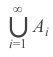is:
 A Z B Q C R D C
Engineering-Mathematics       Set-Theory
Question 86 Explanation:
● In mathematics, there are multiple number sets: the natural numbers N, the set of integers Z, all decimal numbers D, the set of rational numbers Q, the set of real numbers R and the set of complex numbers C.
Z number set:
● Z is the set of integers, ie. positive, negative or zero.
● Example: ..., -100, ..., -12, -11, -10 , ..., -5, -4, -3, -2, - 1, 0, 1, 2, 3, 4, 5, ... 10, 11, 12, ..., 100, ...
● The set N is included in the set Z (because all natural numbers are part of the relative integers).
● The set A consists of Positive, negative and zero numbers
 Question 87
Match the following in List - I and List - II, for a function f :A (a)-(i), (b)-(ii), (c)-(iii) B (a)-(iii), (b)-(ii), (c)-(i) C (a)-(ii), (b)-(i), (c)-(iii) D (a)- (ii), (b)-(iii), (c)-(i)
Engineering-Mathematics       Functions
Question 87 Explanation:
● The function is injective (one-to-one) if each element of the codomain is mapped to by at most one element of the domain. An injective function is an injection. Notationally:
∀ x, x ′ ∈ X , f (x) = f (x ′ ) ⇒ x = x ′
Or, equivalently (using logical transposition),
∀ x, x ′ ∈ X , x = / x ′ ⇒ f (x) = / f (x ′ )
● The function is surjective (onto) if each element of the codomain is mapped to by at least one element of the domain. (That is, the image and the codomain of the function are equal.) A surjective function is a surjection. Notationally:
∀ y ∈ Y , ∃x ∈ X such that y = f (x)
A constant function is a ​ function​ whose (output) value is the same for every input value. For example, the function y(x) =4 is a constant function because the value y(x) is 4 regardless of the input value x
 Question 88
Which of the relations on {0, 1, 2, 3} is an equivalence relation ?
 A { (0, 0) (0, 2) (2, 0) (2, 2) (2, 3) (3, 2) (3, 3) } B { (0, 0) (1, 1) (2, 2) (3, 3) } C { (0, 0) (0, 1) (0, 2) (1, 0) (1, 1) (1, 2) (2, 0) } D { (0, 0) (0, 2) (2, 3) (1, 1) (2, 2) }
Engineering-Mathematics       Relations
Question 88 Explanation:
→ A relation on a set A is called an equivalence relation if it is reflexive, symmetric, and transitive.
1. Reflexivity: f(x) = f(x)
True, as given the same input, a function always produces the same output
2. Symmetry: if f(x) = f(y) then f(y) = f(x)
True, by the definition of equality
3.Transitivity: if f(x) = f(y) and f(y) = f(z) then f(x) = f(z)
True, by the definition of equality
Option-2: { (0,0), (1,1), (2,2), (3,3) }
Has all the properties, thus, is an equivalence relation
Option-1: { (0,0), (0,2), (2,0), (2,2), (2,3), (3,2), (3,3) }
Not reflexive: (1,1) is missing
Not transitive: (0,2) and (2,3) are in the relation, but not (0,3)
Option-3: { (0,0), (0,1) (0,2), (1,0), (1,1), (1,2), (2,0), (2,2), (3,3) }
Not symmetric: (1,2) is present, but not (2,1)
Not transitive: (2,0) and (0,1) are in the relation, but not (2,1)
Option-4: Similarly, option-4 also not TRUE
 Question 89
Which of the following is an equivalence relation on the set of all functions from Z to Z ?
 A { (f , g ) | f (x) − g (x) = 1 ∀ x ∈ Z } B { (f , g ) | f (0) = g (0) or f (1) = g (1)} C { (f , g ) | f (0) = g (1) and f (1) = g (0)} D { (f , g ) | f (x) − g (x) = k f or some k ∈ Z }
Engineering-Mathematics       Relations
Question 89 Explanation:
An equivalence relation is a binary relation that is reflexive, symmetric and transitive.
The relation "is equal to" is the canonical example of an equivalence relation, where for any objects a, b, and c:
a = a (reflexive property),
if a = b then b = a (symmetric property), and
if a = b and b = c then a = c (transitive property)
 Question 90
Which of the following statements is true ?
 A (Z, ≤ ) is not totally ordered B The set inclusion relation ⊆ is a partial ordering on the power set of a set S C (Z, ≠ ) is a poset D The directed graph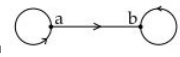is not a partial order
Engineering-Mathematics       Relations
Question 90 Explanation:
Option 1: ( z, ≤ ) is false becauseOption 2: This is TRUE, because the set inclusion relation ⊆ is a partial ordering on the power set of S .
Example: Suppose the set S = { a, b } , the maximum possibilities are { φ, a , b , a b}Option 3: ( z, =) is false, because if violates reflexive relation.
Option 4: FALSE.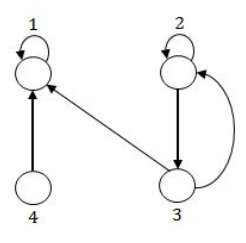Question 91
CMOS is a Computer Chip on the motherboard, which is :
 A RAM B ROM C EPROM D Auxiliary storage
omputer-Organization       CMOS
Question 91 Explanation:
Complementary metal-oxide Semiconductor(CMOS) is a random access memory which stores computer start up information which is used by BIOS.
 Question 92
In RS flip-flop, the output of the flip-flop at time (t+1) is same as the output at time t, after the occurrence of a clock pulse if :
 A S=R=1 B S=0, R=1 C S=1, R=0 D S=R=0
Digital-Logic-Design       Flip-Flops
Question 92 Explanation:
Characteristic table of SR flip-flop where the Next State remains same when S=R=0Question 93
Match the terms in List - I with the options given in List - II :A (a)-(ii), (b)-(i), (c)-(iii) B (a)-(ii), (b)-(iii), (c)-(i) C (a)-(ii), (b)-(i), (c)-(iv) D (a)-(iv), (b)-(ii), (c)-(i)
Digital-Logic-Design       Match-The-Following
Question 93 Explanation:
Decoder:Question 94
What does the following logic diagram represent ?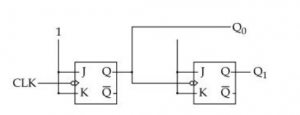A Synchronous Counter B Ripple Counter C Combinational Circuit D Mod 2 Counter
Digital-Logic-Design       Combinational-Circuits
Question 94 Explanation:
→ A ripple counter is an asynchronous counter where only the first flip-flop is clocked by an external clock.
→ All subsequent flip-flops are clocked by the output of the preceding flip-flop. Asynchronous counters are also called ripple-counters because of the way the clock pulse ripples it way through the flip-flops.
→ The MOD of the ripple counter or asynchronous counter is 2​ n​ if n flip-flops are used.Question 95
The hexadecimal equivalent of the binary integer number 110101101 is :
 A D24 B 1 B D C 1 A E D 1 A D
Digital-Logic-Design       Number-System
Question 95 Explanation:
(110101101) 2 = ( ? ) 16 2 4 = 1 6
So, 4-bits in binary will represent one integer in Hexadecimal.
So,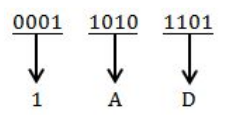Question 96
​ Perform the following operation for the binary equivalent of the decimal numbers (-14)10+(15)10 The solution in 8 bit representation is :
 A 11100011 B 00011101 C 10011101 D 11110011
Digital-Logic-Design       Number-System
Question 96 Explanation:
(− 1 4) 10 + (− 1 5) 10 = (− 2 9) 10(29) 10 = (11101) 2
(29) 10 in 8-bit representation = (00011101) 2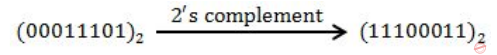(− 2 9) 10 = (00011101) 2
 Question 97
Match the items in List - I and List - II :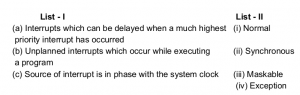A (a)-(ii), (b)-(i), (b)-(iv) B (a)-(ii), (b)-(iv), (b)-(iii) C (a)-(iii), (b)-(i), (b)-(ii) D (a)-(iii), (b)-(iv), (b)-(ii)
Digital-Logic-Design       Match-the-following
Question 97 Explanation:
Maskable Interrupt : Maskable Interrupts are those which can be masked/delayed when a higher priority interrupt occurs.
Exception : Exception are the unplanned interrupts that occur while executing a program. Divide by zero is an example of Exception.
Synchronous: Synchronous clocks will regularly synchronize their time with a master clock. The connection can be direct (wired), or over the air (radio, wifi or similar). The connections can be at a regular intervals, on demand or a mix.
 Question 98
Which of the following mapping is not used for mapping process in cache memory?
 A Associative mapping B Direct mapping C Set-Associative mapping D Segmented - page mapping
Computer-Organization       Cache-Memory
Question 98 Explanation:
Segmented - page mapping is the mapping not used for mapping process in cache memory
 Question 99
Simplify the following using K-map : F (A, B, C, D) = Σ (0, 1, 2, 8, 9, 12, 13) d (A, B, C, D) = Σ (10, 11, 14, 15) d stands for don’t care condition.
 ABC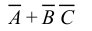DDigital-Logic-esign       K-Map
Question 99 Explanation:Question 100
​ In 8085 microprocessor, what is the output of following program ? LDA 8000H MVI B, 30H ADD B STA 8001H
 A Read a number from input port and store it in memory B Read a number from input device with address 8000H and store it in memory at location 8001H C Read a number from memory at location 8000H and store it in memory location 8001H D Load A with data from input device with address 8000H and display it on the output device with address 8001H
Computer-Organization       Microprocessor
Question 100 Explanation:
→ 1st instruction "LDA 8000H" transfers data from memory location 8000H to Accumulator
→ 2nd instruction "MVI B, 30H" moves 30H to register B.
→ 3rd instruction "ADD B" adds contents of B with Accumulator and stores it back to Accumulator. So basically the contents of Accumulator are incremented by 30H.
→ 4th instruction "STA 8001H" stores the contents of Accumulator in memory location 8001H.
As none of the choices include the 'addition operation', we need to choose appropriate option from the given options.
→ Option 4 mentions about loading the content from 8000H to accumulator A. Hence option 4 is more appropriate.
There are 100 questions to complete.
PHP Code Snippets Powered By : XYZScripts.com